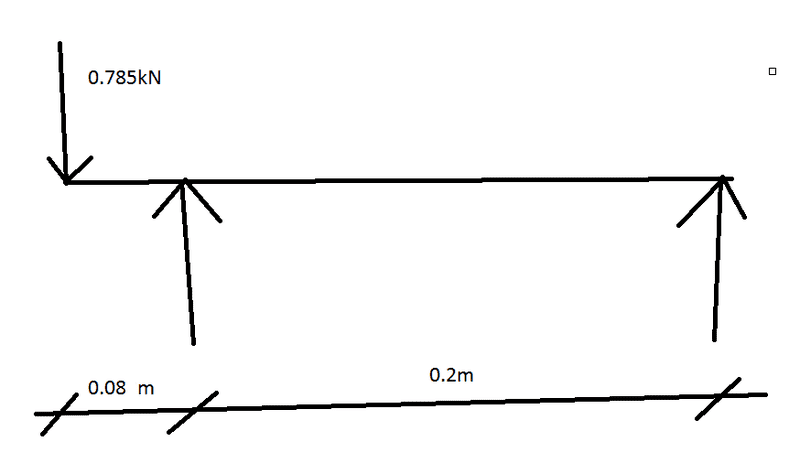# Simple bending moment calculation

Hi,

Really need some help with figuring out the max bending moment in the diagram shown below (I'm aware this is extremely simple but time and stress appear to have the better of me, so any help is appreciated)I think I've worked out the reactions at each support by doing m=wL as the following

0.785 x 0.08 = 0.63
0.785 x 0.28 = 0.22

I'm also aware that I could be completely wrong with what I've just put above.

SOS

Nidum
Gold Member
The beam would probably just tip over with the forces acting in the directions shown .

We need to understand the actual loading system more clearly before calculating bending moments .

Can you post a sketch showing the actual mechanical assembly that your line drawing represents ?

I don't think the reactions are correct. As drawn, ΣM = 0 = 0.2R + 0.785(0.08) : R = - 0.314 kN : The "-" sign simply implies you have the right reaction, R, acting the opposite direction. ΣF = 0 = - 0.785 - 0.314 + L : The left reaction, L = 1.099 kN : Given the reactions you should be able to calculate the moment.
Draw shear and moment diagrams.

•Wilson123
I don't think the reactions are correct. As drawn, ΣM = 0 = 0.2R + 0.785(0.08) : R = - 0.314 kN : The "-" sign simply implies you have the right reaction, R, acting the opposite direction. ΣF = 0 = - 0.785 - 0.314 + L : The left reaction, L = 1.099 kN : Given the reactions you should be able to calculate the moment.
Draw shear and moment diagrams.

Thanks a lot for your help.

Draw a shear diagram first.